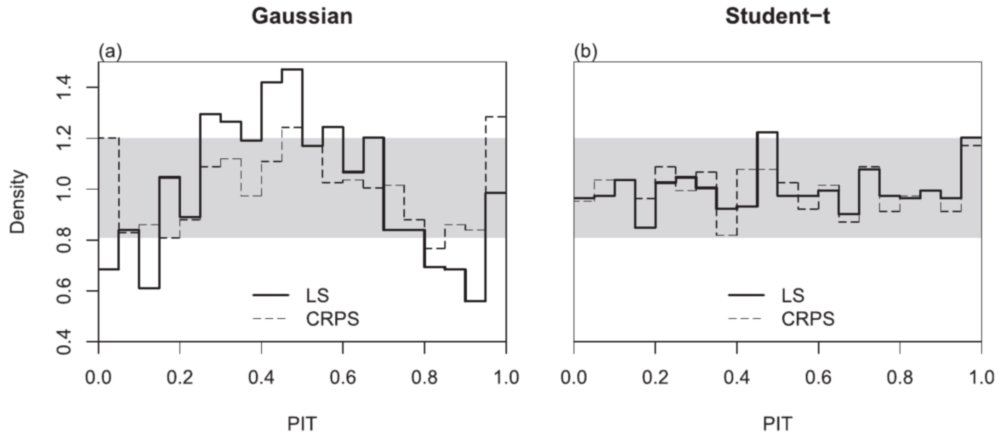Want to share your content on R-bloggers? click here if you have a blog, or here if you don't.

In a new paper in Monthly Weather Review, minimum CRPS and maximum likelihood estimation are compared for fitting heteroscedastic (or nonhomogenous) regression models under different response distributions. Minimum CRPS is more robust to distributional misspecification while maximum likelihood is slightly more efficient under correct specification. An R implementation is available in the crch package.

### Citation

Manuel Gebetsberger, Jakob W. Messner, Georg J. Mayr, Achim Zeileis (2018). “Estimation Methods for Nonhomogeneous Regression Models: Minimum Continuous Ranked Probability Score versus Maximum Likelihood.” Monthly Weather Review. 146(12), 4323-4338. doi:10.1175/MWR-D-17-0364.1

### Abstract

Nonhomogeneous regression models are widely used to statistically postprocess numerical ensemble weather prediction models. Such regression models are capable of forecasting full probability distributions and correcting for ensemble errors in the mean and variance. To estimate the corresponding regression coefficients, minimization of the continuous ranked probability score (CRPS) has widely been used in meteorological postprocessing studies and has often been found to yield more calibrated forecasts compared to maximum likelihood estimation. From a theoretical perspective, both estimators are consistent and should lead to similar results, provided the correct distribution assumption about empirical data. Differences between the estimated values indicate a wrong specification of the regression model. This study compares the two estimators for probabilistic temperature forecasting with nonhomogeneous regression, where results show discrepancies for the classical Gaussian assumption. The heavy-tailed logistic and Student?s t distributions can improve forecast performance in terms of sharpness and calibration, and lead to only minor differences between the estimators employed. Finally, a simulation study confirms the importance of appropriate distribution assumptions and shows that for a correctly specified model the maximum likelihood estimator is slightly more efficient than the CRPS estimator.

### Software

https://CRAN.R-project.org/package=crch

The function `crch()` provides heteroscedastic (or nonhomogenous) regression models of `"gaussian"` (i.e., normally distributed), `"logistic"`, or `"student"` (i.e., t-distributed) response variables. Additionally, responses may be censored or truncated. Estimation methods include maximum likelihood (`type = "ml"`, default) and minimum CRPS (`type = "crps"`). Boosting can also be employed for model fitting (instead of full optimization). CRPS computations leverage the excellent scoringRules package.

### Illustration

The plots below show histograms of the PIT (probability integral transform) for various nonhomogenous regression models yielding probabilistic 1-day-ahead temperature forecasts at an Alpine site (Innsbruck). When the probabilistic forecasts are perfectly calibrated to the actual observations the PIT histograms should form a straight line at density 1. The gray area illustrates the 95% consistency interval around perfect calibration – and binning is based on 5% intervals.When a normally distributed or Gaussian response is assumed (left panel), it is shown that the maximum-likelihood model (solid line) is not well calibrated as the tails are not heavy enough. (The legend denotes this “LS” because maximizing the likelihood is equivalent to minimizing the so-called log-score.) In contrast, the minimum-CRPS model is reasonably well calibrated.

When assuming a Student-t response (right panel) there is little deviation between both estimation techniques and both are well-calibrated.

Thus, the source of the differences between CRPS- and ML-based estimation with a Gaussian response comes from assuming a distribution whose tails are not heavy enough. In this situation, minimum-CRPS yields the somewhat more robust model fit while both estimation techniques lead to very similar results if a more suitable response distribution is adopted. In the latter case ML is slightly more efficient than minimum-CRPS.